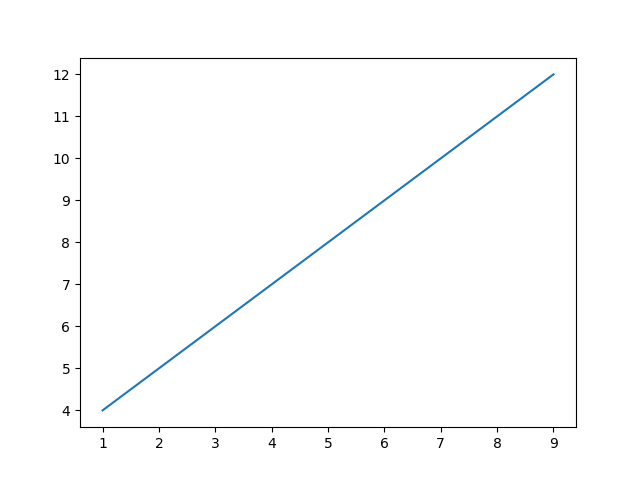## 15 Feb Matplotlib – Line Graph

To draw a line graph in Matplotlib, use the plot() method. By default, plot() creates a line. The points used are the x and y coordinates of a line. Let us see an example wherein we will plot the x and y points:

OutputIf you liked the tutorial, spread the word and share the link and our website Studyopedia with others.